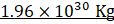### Question 15. Solve the following example. The mass of the earth is 6 x 1024 kg. The distance between the earth and the Sun is 1.5x 1011 m. If the gravitational force between the two is 3.5 x 1022 N, what is the mass of the Sun?

Question 15.

Solve the following example.

The mass of the earth is 6 x 1024 kg. The distance between the earth and the Sun is 1.5x 1011 m. If the gravitational force between the two is 3.5 x 1022 N, what is the mass of the Sun?

Use G = 6.7 x 10-11 N m2 kg-2

Gravitational force of attraction between two bodies of masses m1 and m2separated by a distance of R is given by;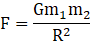G = Gravitational constant

Putting values of m1 =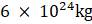;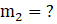;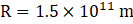.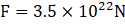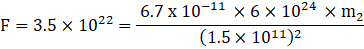⇒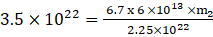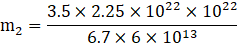Since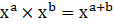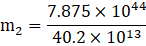Since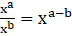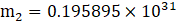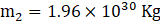.

Therefore mass of the sun is